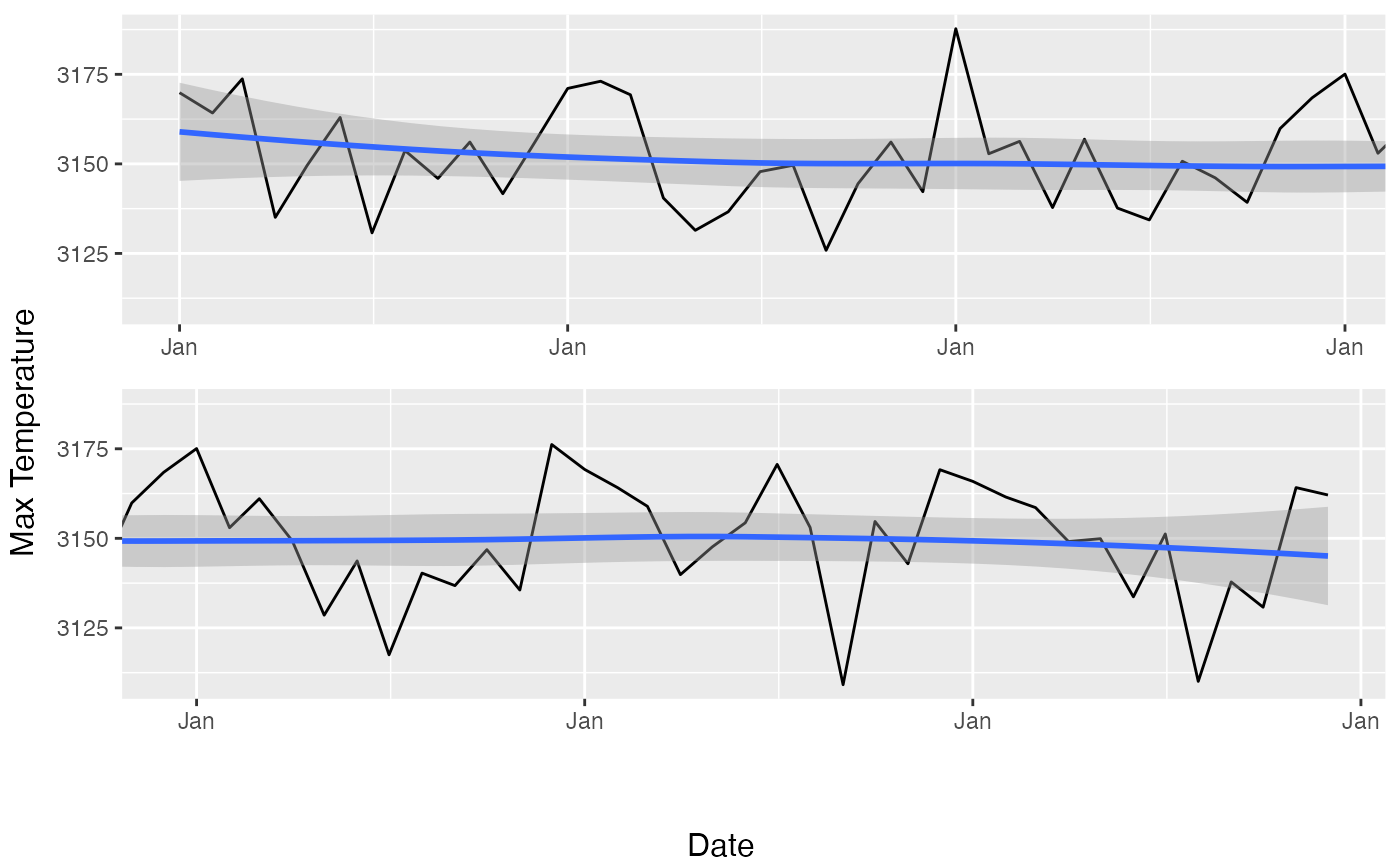Long timeseries can be compressed to the point of being unreadable when plotted on a page. This function takes a ggplot object of a timeseries and divides it into panels so that the time dimension gets stretched for better readability.

DivideTimeseries(g, x, n = 2, xlab = "x", ylab = "y")

## Arguments

g

ggplot object

x

The vector that was used in g for the x axis (must be of class Date)

n

Number of panels

xlab

x axis label

ylab

y axis label

## Value

Draws a plot.

Other ggplot2 helpers: MakeBreaks(), WrapCircular(), geom_arrow(), geom_contour2(), geom_contour_fill(), geom_label_contour(), geom_relief(), geom_streamline(), guide_colourstrip(), map_labels, reverselog_trans(), scale_divergent, scale_longitude, stat_na(), stat_subset()

## Examples

library(ggplot2)
library(data.table)
gdata <- geopotential[lat == -30 & lon == 0]
g <- ggplot(gdata, aes(date, gh)) +
geom_line() +
geom_smooth() +
scale_x_date(date_breaks = "1 year", date_labels = "%b")
DivideTimeseries(g, gdata$date, n = 2, "Date", "Max Temperature") #> Warning: 'DivideTimeseries' is deprecated, use ggwrap instead (https://github.com/wilkox/ggwrap). #> geom_smooth() using method = 'loess' and formula = 'y ~ x' #> Error in pl$grobs[[leg]] :
#>   attempt to select less than one element in get1index
#> Warning: The <scale> argument of guides() cannot be FALSE. Use "none" instead as
#> of ggplot2 3.3.4.
#> ℹ The deprecated feature was likely used in the metR package.
#>   Please report the issue at <https://github.com/eliocamp/metR/issues>.
#> geom_smooth() using method = 'loess' and formula = 'y ~ x'
#> geom_smooth() using method = 'loess' and formula = 'y ~ x'